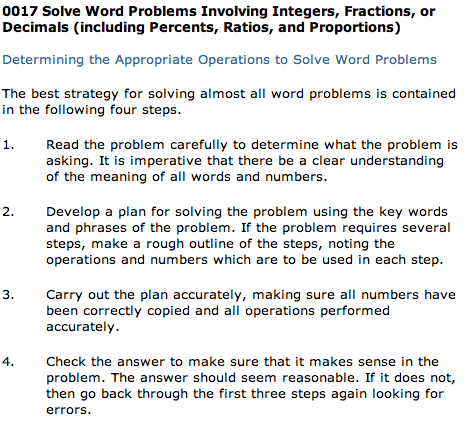Welcome, Student Name | Student Demo | Buy Now

 Contents Mathematics (98)0016 Number Concepts and Computation Skills0017 Word Problems Involving Integers, Fractions, or DecimalsSolving Word ProblemsWord Problems Involving Integers, Fractions, Decimals, Percents, Ratios, and Proportions0018 Interpret Information from a Graph, Table, or Chart0019 Probability and Statistics0020 Graph Numbers or Number Relationships0021 One- and Two-Variable Equations0022 Word Problems Involving One and Two Variables0023 Algebraic Expressions0024 Problems Involving Geometric Figures0025 Apply Reasoning SkillsPractice Math Tests Main Menu Exit Student Demo Buy Now for \$39.95 and begin using the entire course in less than two minutes